World-class research. Ultimate impact.
More on impact ›

Frontiers in ICTOriginal Research ARTICLE

Front. ICT, 30 September 2016 | https://doi.org/10.3389/fict.2016.00021

Monocular, Boundary-Preserving Joint Recovery of Scene Flow and Depth

• 1Institut National de la Recherche Scientifique (INRS-EMT), Montreal, QC, Canada
• 2Ecole de Technologie Superieure (ETS), Montreal, QC, Canada

Variational joint recovery of scene flow and depth from a single image sequence, rather than from a stereo sequence as others required, was investigated in Mitiche et al. (2015) using an integral functional with a term of conformity of scene flow and depth to the image sequence spatiotemporal variations, and L2 regularization terms for smooth depth field and scene flow. The resulting scheme was analogous to the Horn and Schunck optical flow estimation method, except that the unknowns were depth and scene flow rather than optical flow. Several examples were given to show the basic potency of the method: it was able to recover good depth and motion, except at their boundaries because L2 regularization is blind to discontinuities which it smooths indiscriminately. The method that we study in this paper generalizes to L1 regularization the formulation of Mitiche et al. (2015) so that it computes boundary-preserving estimates of both depth and scene flow. The image derivatives, which appear as data in the functional, are computed from the recorded image sequence also by a variational method, which uses L1 regularization to preserve their discontinuities. Although L1 regularization yields non-linear Euler–Lagrange equations for the minimization of the objective functional, these can be solved efficiently. The advantages of the generalization, namely, sharper computed depth and three-dimensional motion, are put in evidence in experimentation with real and synthetic images, which shows the results of L1 versus L2 regularization of depth and motion, as well as the results using L1 rather than L2 regularization of image derivatives.

1. Introduction

Scene flow is the three-dimensional (3D) motion field of the visible environmental surfaces projected on the image domain: at each image point, scene flow is the 3D velocity of the corresponding environmental surface point. It is the time derivative of 3D position. As such, it is a function of both depth and optical flow (Longuet-Higgins and Prazdny, 1981). Scene flow computation has been the focus of several recent studies (Vedula et al., 2005; Huguet and Devernay, 2007; Pons et al., 2007; Wedel et al., 2008; Mitiche and Aggarwal, 2013; Vogel et al., 2013). It is a typical inverse problem best stated by variational formulations (Mitiche and Aggarwal, 2013). Basic variational statements use L2 (Tikhonov) regularization. This regularization, which imposes smoothness on the solution, yields linear terms in the Euler–Lagrange equations. This is the case with the scheme described in Mitiche et al. (2015) and of which we describe a generalization in this paper. The scheme improved significantly on others because it needed a single image sequence rather than a stereo stream like other methods. Also, it formulated the problem using an integral functional of two terms: a data fidelity term to constrain the computed scene flow to conform to the image sequence spatiotemporal derivatives and L2 regularization terms to constrain the computed scene flow and depth to be smooth, which led to linear Euler–Lagrange equations for the minimization of the objective functional, much like in the Horn and Schunck optical flow formulation (Horn and Schunk, 1981), except that it involved depth and scene flow rather than optical flow. However, L2 regularization blurs the computed scene flow and depth boundaries because it imposes their smoothness everywhere. In general, some form of boundary-preserving regularization is necessary. This is particularly true when environmental objects move independently relative to the viewing system. The variation of motion and structure in such a case can be sharp and significant at these objects occluding boundaries, and therefore, accuracy would require that they be preserved by the regularization operator.

Boundary-preserving recovery of scene flow and depth can be specified in various ways (Mitiche and Aggarwal, 2013). For example, instead of the L2 norm, one can use the Aubert et al. function (Aubert et al., 1999; Aubert and Kornprobst, 2002) or the L1 norm. We can also inhibit smoothing across boundaries by joint 3D motion estimation and segmentation (Mitiche and Sekkati, 2006). In this follow-up study of our previous investigation (Mitiche et al., 2015), we apply the L1 regularization. There are three basic reasons for this choice: (1) the ability of the L1 constraint to preserve sharp boundaries while penalizing oscillations. This is well suited for “blocky images” which tend to be smooth inside regions, which have sharp significant boundaries, as is, in general, typical of motion fields; (2) in practice, it can be implemented by computationally efficient approximations without affecting the accuracy of results in a noticeable way; and (3) there is a significant body of literature in its support, particularly in image restoration (Vogel, 2002).

Recovery of scene flow and depth uses the image sequence spatiotemporal derivatives. The study in Mitiche et al. (2015) computed derivatives by a variational formulation, which used an anti-differentiation data fidelity term, and L2 regularization. The anti-differentiation term expressed the fact that an image derivative is a function which when integrated gives the image. The present study uses the same anti-differentiation data fidelity term but substitutes L1 regularization for L2 in order, first, to be consistent with the use of L1 regularization of scene flow and depth and, second, to have the evaluation of the derivatives account for their discontinuities.

We conducted several experiments with real and synthetic data to verify the validity and efficiency of the scheme. We show comparative results that put in evidence the improvements one can obtain by using L1 rather than L2 regularization of depth and motion, as well as by evaluating the image derivatives with L1 rather than L2 regularization.

The remainder of this paper is organized as follows: Section 2 develops the objective functional, and Section 3 describes its minimization. Section 4 shows how the image derivatives are computed. The validation experiments, using synthetic and real image sequences, are described in Section 5. Section 6 contains a conclusion.

2. Formulation

Let I: (x,y,t) → I(x,y,t) be an image sequence, where (x,y) are the spatial coordinates on the bounded image domain Ω, and tR+ designates time. Let (X,Y,Z) be the coordinates of a point P in space and (x,y) the coordinates of its projection. The coordinate system and the imaging geometry are shown in Figure 1. Let U, V, and W be the scene flow coordinate functions. The scene flow and depth linear gradient constraint (Mitiche et al., 2015), which relates the scene flow coordinates U, V, and W and depth Z to the spatiotemporal image is

$fIxU+fIyV−(xIx+yIy)W+ItZ=0,$

where Ix, Iy, and It are the image spatiotemporal derivatives, Z designates depth (Figure 1), and $(U,V,W)=(dXdt,dYdt,dZdt)$ is the scene flow vector at P. This is a homogeneous linear equation in the variables of scene flow and depth. The homogeneity results from the aperture problem. Multiplication of motion and depth (and structure thereof) by a constant (scale) maintains the equation integrity. One can remove this uncertainty of scale by choosing the depth to be relative to the frontoparallel plane Z = Z0, for some positive depth Z0 (Mitiche et al., 2015). Therefore, equation (1) becomes:

$fIxU+fIyV−(xIx+yIy)W+It(Z−Z0)+ItZ0=0$
FIGURE 1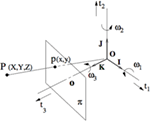Figure 1. The viewing system is represented by a Cartesian coordinate system (O; X,Y,Z) and central projection through the origin. The Z-axis is the depth axis. The image plane π is orthogonal to the Z-axis at distance f, the focal length, from O.

For notational convenience and economy, we will reuse the symbol Z for depth relative to the frontoparallel pane Z = Z0, in which case we can write equation (2) as

$fIxU+fIyV−(xIx+yIy)W+ItZ+ItZ0=0$

In the formulation of Mitiche et al. (2015), scene flow and relative depth resulted from the minimization of the following L2 smoothness regularization functional:

$E(U,V,W,Z|I)=12∫Ω(fIxU+fIyV−(xIx+yIy)W+ItZ+ItZ0)2dxdy+α2∫Ω(∥∇U∥2+∥∇V∥2+∥∇W∥2)dxdy+β2∫Ω∥∇Z∥2dxdy,$

where α and β scale the relative contribution of the terms of the functional. For boundary preservation, we replace the L2 norm regularization term of each variable by an L1 norm term, namely: $∫Ω∥∇Q∥dxdy=∫ΩQx2+Qy212dxdy$, where Q ∈ {U, V, W, Z}. Therefore, the objective functional is

$E(U,V,W,Z|I)=12∫Ω(fIxU+fIyV−(xIx+yIy)W+ItZ+ItZ0)2dxdy+α2∫Ω((Ux2+Uy2)12+(Vx2+Vy2)12+(Wx2+Wy2)12)dxdy+β2∫Ω(Zx2+Zy2)12dxdy,$

3. Optimization

The Euler–Lagrange equations corresponding to functional equation (5) are

$fIx(fIxU+fIyV+(−xIx−yIy)W+ItZ+ItZ0)−α∂∂xUxUx2+Uy212−α∂∂yUyUx2+Uy212=0fIy(fIxU+fIyV+(−xIx−yIy)W+ItZ+ItZ0)−α∂∂xVxVx2+Vy212−α∂∂yVyVx2+Vy212=0(xIx+yIy)(fIxU+fIyV−(xIx+yIy)W+ItZ+ItZ0)+α∂∂xWxWx2+Wy212−α∂∂yWyWx2+Wy212=0It(fIxU+fIyV+(−xIx−yIy)W+ItZ+ItZ0)−β∂∂xZxZx2+Zy212−β∂∂yZyZx2+Zy212=0,$

with the Neumann boundary conditions:

$∂U∂n=0,∂V∂n=0,∂W∂n=0,∂Z∂n=0,$

where $∂∂n$ is the differentiation operator in the direction of the normal n of the boundary ∂Ω of Ω.

Were it not for the denominator of the regularization terms in equation (6), the equations would be linear. It is common in numerical analysis to solve such systems of equations using an iterative method where the non-linear terms are evaluated at the preceding iteration, in which case they are treated as data at the current iteration, and the equations to solve are linear. In our case, we alternate the following two steps at the current k-th iteration.

Step 1: this step accounts of the non-linearity of the obtained Euler–Lagrange equations and consists of updating the denominators of the regularization terms in equation (6) at each grid point:

$g(Qk)=1Qxk−12+Qyk−12+ε12,Q∈{U,V,W,Z}$

where ε is a small positive value whose purpose is to remedy the non-differentiability of the Euclidean norm at the origin, without affecting the computational outcome in a noticeable way. Notice that the pointwise updates in equation (8) have a computational complexity that grows linearly with respect to N (the image size): the complexity is N multiplied by the fixed complexity of 4 updates of the form in equation (8).

Step 2: with pointwise coefficients g(Qk)(Q ∈ {U, V, W, Z}) fixed, this step is an update (iteration k) of variables {U, V, W, Z} for solving the following linear system:

$fIx(fIxU+fIyV+(−xIx−yIy)W+ItZ+ItZ0)−αg(Uk)∇2U=0fIy(fIxU+fIyV+(−xIx−yIy)W+ItZ+ItZ0)−αg(Vk)∇2V=0(xIx+yIy)(fIxU+fIyV−(xIx+yIy)W+ItZ+ItZ0)−αg(Wk)∇2W=0It(fIxU+fIyV+(−xIx−yIy)W+ItZ+ItZ0)−βg(Zk)∇2Z=0,$

The four equations of equation (9) are written for each point of image domain Ω. Let Ω be discretized via a unit-spacing grid, and let the grid points be indexed by the integers {1, 2, …, N}. The pixel numbering is according to the lexicographical order, i.e., by scanning the image top-down and left to right. If the image is of size n × n, then N = n2. Let a = fIx, b = fIy, c = −(xIx + yIy), and d = It.

For all grid point indices i ∈ {1, 2, …, N}, a discrete approximation of the linear system (equation (9)) is

$(S)(ai2+αgi(Uk)ni)Ui+aibiVi+aiciWi+aidiZi−αgi(Uk)∑j∈NiUj=−aidiZ0biaiUi+(bi2+αgi(Vk)ni)Vi+biciWi+bidiZi−αgi(Vk)∑j∈NiVj=−bidiZ0ciaiUi+cibiVi+(ci2−αgi(Wk)ni)Wi+cidiZi−αgi(Wk)∑j∈NiWj=−cidiZ0diaiUi+dibiVi+diciWi+(di2+βgi(Zk)ni)Zi−βgi(Zk)∑j∈NiZj=−di2Z0,$

where (Ui, Vi, Wi, Zi) = (U, V, W, Z)i is the scene flow vector at grid point i; ai, bi, ci, and di are the values at i of a, b, c, and d, respectively, gi(Qk), Q ∈ {U, V, W, Z}, are the pointwise updates of equation (8) evaluated at i, and Ni is the set of indices of the neighbors of i. For the 4-neighborhood, ni = card(Ni) = 4 for points interior to the discrete image domain, and ni < 4 for boundary image points. Laplacian ▽2Q in the Euler–Lagrange equations (Q ∈ {U, V, W, Z}) has been discretized as $14∑j∈Ni(Qj−Qi)$, with α (respectively β) absorbing the factor 1/4.

Let q = (q1, …, q4N)tR4N be the vector with coordinates q4i−3 = Ui, q4i−2 = Vi, q4i−1 = Wi, q4i = Zi, i ∈ {1, …, N}, and r = (r1, …, r4N)tR4N the vector with coordinates r4i−3 = −aidiZ0, r4i−2 = −bidiZ0, r4i−1 = −cidiZ0, and $r4i=−di2Z0$, i ∈ {1, …, N}. System (S) of linear equations can be written in a matrix form as

$Aq=r$

where A is the 4N × 4N matrix with elements $A4i−3,4i−3=ai2+αgi(Uk)ni$; $A4i−2,4i−2=bi2+αgi(Vk)ni$; $A4i−1,4i−1=ci2+αgi(Wk)ni$; $A4i,4i=di2+βgi(Zk)ni$; A4i−3,4i−2 = A4i−2,4i−3 = aibi; A4i−3,4i−1 = A4i−1,4i−3 = aici; A4i−3,4i = A4i,4i−3 = aidi; A4i−2,4i−1 = A4i−1,4i−2 = bici; A4i−2,4i = A4i,4i−2 = bidi; A4i−1,4i = A4i,4i−1 = cidi; for all i ∈ {1, …, N}, and A4i−3,4j−3 = −αgi(UK); A4i−2,4j−2 = −αgi(Vk); A4i−1,4j−1 = −αgi(Wk); and A4i,4j = −βgi(Zk), for all i, j ∈ {1, …, N} such that jNi, all other elements being equal to zero. This is a large-scale sparse system of linear equations, which can be solved by iterative updates for sparse matrices (Ciarlet, 1994; Stoer and Bulirsch, 2002). It is easy to prove that symmetric matrix A is positive definite (PD). This means that a fast solution of equation (10) can be obtained by convergent 4 × 4 block-wise Gauss–Seidel updates. To show that A is PD, it suffices to perform some algebraic manipulations to write qtAq for all qR4N, q ≠ 0, as follows:

$qtAq=∑i=1N(aiUi+biVi+ciWi+diZi)2+α∑i=1N∑j∈Ni;j>igi(Uk)(Ui−Uj)2+gi(Vk)(Vi−Vj)2+gi(Wk)(Wi−Wj)2+β∑i=1N∑j∈Ni;j>igi(Zk)(Zi−Zj)2>0$

The positive definiteness of A implies that iterative pointwise and block-wise Gauss–Seidel and relaxation updates for system (equation (10)) converge (Ciarlet, 1994; Stoer and Bulirsch, 2002). For a 4 × 4 block division of A, the Gauss–Seidel update (iteration k) for each grid point i ∈ {1, …, N} is

$(ai2+αgi(Uk)ni)Uik+1+aibiVik+1+aiciWik+1+aidiZik+1=−aidiZ0+αgi(Uk)∑j∈Ni;jiUjkbiaiUik+1+(bi2+αgi(Vk)ni)Vik+1+biciWik+1+bidiZik+1=−bidiZ0+αgi(Vk)∑j∈Ni;jiVjkciaiUik+1+cibiVik+1+(ci2+αgi(Wk)ni)Wik+1+cidiZik+1=−cidiZ0+αgi(Wk)∑j∈Ni;jiWjkdiaiUik+1+dibiVik+1+diciWik+1+(di2+βgi(Zk)ni)Zik+1=−di2Z0+βgi(Zk)∑j∈Ni;jiZjk,$

For each point I ∈ {1, …, N}, we solve a 4 × 4 linear system of equations, which can be done efficiently by a singular value decomposition (SVD) (Forsythe et al., 1977). The computational complexity of this step grows linearly with respect to N: the complexity is N multiplied by the fixed complexity of a 4 × 4 SVD.

3.1. Computational Load: L1 vs. L2

Although L1 regularization yields non-linear Euler–Lagrange equations for the minimization of our objective functional, these can be solved efficiently via the two-step scheme that we proposed above. The pointwise updates in equation (8) account for the non-linearity of the L1 model (Step 1) and are an additional computational load in comparison to L2 regularization. However, these updates have a complexity that grows linearly with respect to N (image size). Therefore, they do not increase the computational time substantially; see the computational times in Table 1. Step 2 has a computational complexity that is similar to the L2 regularization of Mitiche et al. (2015): in both cases, we have block-wise (4 × 4) and relaxation updates for a large-scale sparse system of linear equations, with a symmetric positive definite matrix A. The complexity of each iteration is N multiplied by the fixed complexity of a 4 × 4 singular value decomposition, and the positive definiteness of A implies that the block-wise updates converge.

TABLE 1

Table 1 reports the computation (CPU) times for both L1 and L2 regularizations, in the case of four different test images of different sizes. Columns 2 and 3 contain the overall CPU times. The third column gives the CPU time for the block-wise SVD updates solving a large-scale sparse system. These SVD computations occur in both L2 and L1 formulations (Step 2). The last column reports the CPU times for the pointwise updates in equation (8). These updates appear only in the L1 formulation (Step 1). The simulations were run on an Intel i7-4500U Processor (4M Cache, up to 3.00 GHz), using MATLAB (2014b version).

4. Partial Derivatives

In computer vision, image derivatives are often approximated by locally averaged finite differences to lessen the impact of noise (Horn and Schunk, 1981; Marshall, 2005; Periaswamy and Farid, 2006; Sun et al., 2010; Heinrich et al., 2012; Mitiche et al., 2015). However, such fixed-support low-pass filtering does not generally fit the noise profile and can, therefore, be ineffective. A more effective way is to state differentiation as a spatially regularized variational problem, as was done in Mitiche et al. (2015). The process advocated in Mitiche et al. (2015) looked at the derivative of an image as a function which, when integrated, gives the image function. Consequently, the objective functional contained an anti-differentiation data term, which evaluates the conformity of a derivative to the image by constraining the integration of this derivative to produce the image. The functional also contained an L2 regularization term. In this paper, we investigate a generalization, which accounts for derivative discontinuities using an L1 regularization term because L2 regularization constrains the image derivatives to be smooth everywhere and, as a result, would adversely blur their boundaries.

4.1. L1 Regularized Differentiation

The partial derivatives Ix and Iy of an image will be estimated using an anti-differentiation characterization. The method for Ix will be explained in this section. The derivative Iy is computed by the same scheme applied to the transposed image. Since only two images are available along the time axis, the temporal derivative will be estimated using the Horn and Schunck definition of the temporal derivative (Horn and Schunk, 1981). Let f designate an approximation of the derivative. Recall that in Mitiche et al. (2015), the derivative was computed by minimizing the following functional with respect to f :

$E(f)=12∫Ω∥Df−I∥2+γ∥∇f∥2dxdy,$

where D is the anti-differentiation operator, γ is a positive constant, and ▽f is the spatial gradient of f. The integral operator of anti-differentiation D is defined by

$Df(x,y)=∫0xf(z,y)dz$

To generalize this formulation to preserve boundaries, we will replace the L2 regularization term in equation (13) by an L1 term: $∫Ωfx2+fy212dxdy$. The objective functional becomes

$E(f)=12∫Ω∥Df−I∥2+γ∫Ω(fx2+fy2)12dxdy,$

The corresponding Euler–Lagrange equations are

$D∗(Df−I)−γ∂∂xfxfx2+fy212−γ∂∂yfyfx2+fy212=0,$

where D* is the adjoint operator of D given by, assuming Ω = [0,l] × [0,l], $D∗f(x,y)=∫xlf(z,y)dz$.

Non-linearity occurs in the regularization terms of equation (23). As done in the previous section, we can, in practice, and without affecting in any significant way subsequent processing, extend differentiability to the origin by replacing the denominators by $fx2+fy2+ε12$, for some small positive ε. Also, and as we have done in the previous section, solve equation (16) iteratively, by evaluating, at each iteration k, the non-linear terms at the preceding iteration k − 1. More precisely, we have, after initialization, the following equation at iteration k:

$D∗(Dfk−I)−γfxk−12+fyk−12+ε12∇2fk=0$

As in Mitiche et al. (2015), discretization of equation (17) yields a large-scale sparse system of linear equations which can be solved by the Gauss–Seidel method.

4.2. Example

We apply the scheme to compute the derivatives of the noisy (Gaussian white noise, SNR = 1) synthetic chessboard image of Figure 2, which illustrates that L1 regularized differentiation outperforms both the local finite-difference definition and L2 regularized differentiation. A quantitative evaluation can be done by computing the MSE (mean squared error) and the SDE (standard deviation of error). Table 2 lists the results. Derivatives are in gray level (0–255 range) per pixel. The top part of the table gives the measurements as obtained from the whole image. The values listed in the bottom part of the table come from the vicinity of the image boundaries, using a 5 × 5 window centered on these. The results confirm visual inspection, i.e., that L1 regularization outperforms both L2 regularization and local finite differences.

FIGURE 2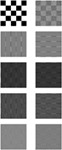Figure 2. Noised chessboard. First row, chessboard image on the left and noised chessboard image on the right (SNR = 1). Second row, ground truth of partial derivatives: Ix on the left and Iy on the right. Third row, estimated partial derivatives using TV regularized differentiation with γ = 1: Ix on the left and Iy on the right. Fourth row, estimated partial derivative using L2 regularized differentiation with γ = 1: Ix on the left Iy on the right. Fifth row, estimated partial derivative using forward difference of Horn and Schunck: Ix on the left and Iy on the right.

TABLE 2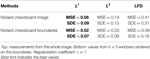Table 2. Differentiation: L1 regularization, L2 regularization, and local finite differences (LFD) applied to noised chessboard image (SNR = 1) and evaluated using mean squared error (MSE) and standard deviation error (SDE).

5. Experimental Results

In this section, we expose various experiments showing the application of the described method to synthetic and real image sequences. In addition to displaying various experimental examples, we present a comparative and quantitative analysis that highlights the positive effects of L1 regularization over the whole image domain, and particularly within motion boundaries. In our experiments, all formulation parameters are determined empirically and distances are measured in pixels. In all the examples, regularized differentiation’s coefficient γ has been fixed equal to 1. As approximated in Sekkati and Mitiche (2007), the focal length of the camera is 600 pixels. The position of the frontoparallel plane Z0 is fixed to 6 × 104 pixels. The initial values of scene flow and depth at each point are set to, respectively, 0 and Z0. Coefficients α and β vary from a sequence to another and are given in the caption of each example.

5.1. Examples

Four image sequences with different characteristics served as samples to test the validity of our scheme and its implementation. Our displays include

• Anaglyphs, which provide a convenient way for subjective appraisal of the recovered object depth. When viewed with chromatic (red–cyan) glasses on good-quality photographic paper or on standard screens, anaglyphs give viewers a strong sense of depth. They are produced from one of the two input images and the computed depth map and are generally better perceived with full color resolution;

• Standard displays using color-coded depth so as to highlight image-depth variations;

• 3D reconstructed objects;

• 3D scene flow vector fields; and

• 2D optical flow fields corresponding to our recovered scene flow. This can serve as an indirect validation of our implementation when the 2D outputs are compared to standard optical flow methods (e.g., the well tested/researched benchmark algorithm of Horn and Schunck).

We provide several figures, each corresponding to an example and organized as follows. The first row includes (from left to right): (a) an anaglyph of the scene structure reconstructed from our method’s output and the first frame of the sequence; (b) a color-coded display of the recovered depth and the used color palette, with depth increasing from bottom (red) to top (purple); and (c) novel viewpoint images of the moving objects in the scene. The second row depicts (from left to right): (a) a view of the obtained scene flow; (b) a projected optical flow corresponding to our estimated scene flow; and (c) optical flow computed directly by the Horn and Schunck algorithm.

The following describes the four image sequences that we used:

• The Marbled-block synthetic image sequence (Figure 3) is taken from the database of KOGS/IAKS Laboratory, Germany. This sequence shows three blocks, two of them are moving. The rightmost block is moving backward to the left, whereas the front-most (smallest) block moves forward to the left. Some aspects of this sequence make its 3D interpretation challenging: the blocks and the floor have a similar macrotexture with weak spatiotemporal intensity variations within the textons. This makes the occluding boundaries of the blocks ill defined at some places. Also, the source of light position with respect to the blocks causes shadows, which move with the blocks.

• The Cylinder and box real sequence (Figure 4), provided in Debrunner and Ahuja (1998), depicts two moving objects, along with a moving background: a cylindrical surface rotating at a velocity of 1° per frame about the vertical axis and moving laterally at an image rate of about 0.15 pixel per frame toward the right, as well as a box translating at approximately 0.30 pixel per frame toward the right. Also, the background is translating to the right (parallel to the box motion) at a rate of about 0.15 pixel per frame. Those motions make 3D interpretation and recovery challenging in this example.

• The Berber real sequence (Figure 5) exhibits a sculpture rotating about the vertical axis and translating forward to the left in a static environment.

• The Pharaohs sequence (Figure 6) shows two moving sculptures in a static environment. The first (leftmost) figurine translates left and forward, whereas the second rotates about a nearly vertical axis to the right.

FIGURE 3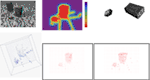Figure 3. Marbled blocks sequence results (better perceived when figures are enlarged on screen). Parameters: α = 6 × 107 and β = 103. First row from left to right: an anaglyph of the structure reconstructed from the method’s output and the first frame of the sequence; a color-coded display of the recovered depth along with the used color palette, with depth increasing from bottom (red) to top (purple); novel viewpoint images of the two moving blocks. Second row: a view of the scene flow vectors; optical flow corresponding to the estimated scene flow; optical flow computed directly by the Horn and Schunck algorithm.

FIGURE 4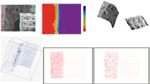Figure 4. Cylinder and box sequence results (better perceived when figures are enlarged on screen). Parameters: α = 6 × 107 and β = 105. First row from left to right: an anaglyph of the structure reconstructed from the method’s output and the first frame of the sequence; a color-coded display of the recovered depth along with the used color palette, with depth increasing from bottom (red) to top (purple); novel viewpoint images of the cylindrical surface and the box. Second row: a view of the scene flow vectors; optical flow corresponding to the estimated scene flow; optical flow computed by the Horn and Schunck algorithm.

FIGURE 5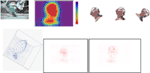Figure 5. Berber figurine sequence results (better perceived when figures are enlarged on the screen). Parameters: α = 6 × 108; β = 5 × 105. First row from left to right: an anaglyph of the structure reconstructed from the method’s output and the first frame of the input image sequence; a color-coded display of the recovered depth along with the used color palette, with depth increasing from bottom (red) to top (purple); novel viewpoint images of the figurine. Second row: a view of the scene flow vectors; optical flow corresponding to the estimated scene flow; optical flow computed by the Horn and Schunck algorithm.

FIGURE 6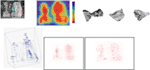Figure 6. Pharaohs figurines sequence (better perceived when figures are enlarged on screen). Parameters: α = 6 × 108; β = × 102. First row from left to right: an anaglyph of the structure reconstructed from the method’s output and the first frame of the input image sequence; a color-coded display of the recovered depth along with the used color palette, with depth increasing from bottom (red) to top (purple); novel viewpoint images of the figurines. Second row: a view of the scene flow vectors; optical flow corresponding to the estimated scene flow; optical flow computed by the Horn and Schunck algorithm.

The examples above support the validity of our scheme and its implementation. The obtained anaglyphs, color-coded depths, and 3D object reconstructions are consistent with the actual structures of the scenes. The displays of the estimated 3D scene flow and the corresponding 2D optical flow are consistent with the real motion of the objects in scenes. Also, the optical flow derived from our scene flow estimation is in line with a direct optical flow computation by the standard Horn and Schunck algorithm. We notice that the obtained fields are more regular and present less noise than those computed directly with Horn and Schunck algorithm. This is mainly due to the use of 3D information and regularized differentiation.

5.2. Comparative Analysis

We report a comprehensive comparative analysis, which demonstrates the benefits of our L1 formulations. In particular, we focus our evaluations on the boundaries of motions and objects, so as to illustrate the boundary-preserving effect of our L1 methods.

We began our comparative analysis by the simple synthetic sequence Squares, which includes two images with known motions. This sequence depicts two overlapping squares in opposite motions, along with a moving background. The motions for these three elements are known: a translation of the rightmost square by (−1, −1) pixels in the downward-left direction, a translation of the leftmost square by (1, 1) pixels in the top-right direction, and downward translation of the background by (0, −1) pixels. To better test the performance of evaluated schemes, we added noise independently to the first and second image. Noise values are from a discretized, shifted, and truncated Gaussian in the interval between 0 and 100 gray levels, within an overall range of [0, 255]. The first row of Figure 7 displays the first (noised) image (left) and the vector-coded ground truth (right). The second row depicts the optical flows, the first (left) obtained from a projection of the L2 regularized scene flow (Mitiche et al., 2015) and the second from our L1 regularized scene flow. In both cases, we estimated image derivatives with a finite-difference regularization based on the standard Horn and Schunck definition (Horn and Schunk, 1981). We refer to these methods as L2HS (left) and L1HS (right). In the third row, we repeated the same experiment using regularized image differentiation instead of the finite differences. On the left, we depict the result using L2 regularization for both scene flow and image-derivative estimations (L2L2) whereas on the right, we show the result for L1 (L1L1). The optical flows resulting from the four methods are consistent with their expected overall appearance. However, we see clearly a difference between the performances of those algorithms.

FIGURE 7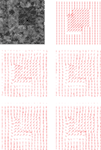Figure 7. Squares synthetic sequence results. First row: the first (noised) image of the sequence (left) and the vector-coded ground truth (right). Second row: the optical flow corresponding to L2HS (left) and L1HS (right). Third row: the optical flow corresponding to L2L2 (left) and L1L1 (right).

Visually, the L1L1 scheme yielded the closest match to the vector-coded ground truth. To support this quantitatively, we added an evaluation based on two standard error measures for optical flow (Baker et al., 2011): average angular error (aae) and endpoint error (epe); see Table 3. The (L1L1) scheme performed better than the other methods.

TABLE 3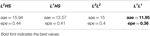Table 3. Performance of L2HS, L1HS, L2L2, and L1L1 algorithms on the noised squares image (SNR = 1.12).

Figure 8 depicts another example for our comparative analysis. It uses the Hydrangea sequence of a real scene from the Middlebury data set (Baker et al., 2011). The sequence shows a rotating flower bouquet within a translating background, and the ground-truth flow of the sequence is given.1 The first row of the figure displays the first of the two input images (left) and the vector-coded ground truth (right). The second row depicts the optical flows, the first (left) obtained from a projection of the L2 regularized scene flow (Mitiche et al., 2015) and the second from our L1 regularized scene flow. In both cases, we estimated image derivatives with a finite-difference regularization based on the standard Horn and Schunck definition (Horn and Schunk, 1981). We refer to these methods as L2HS (left) and L1HS (right). In the third row, we repeated the same experiment using regularized image differentiation instead of the finite differences. On the left, we depict the result using L2 regularization for both scene flow and image-derivative estimations (L2L2) whereas on the right, we show the result for L1 (L1L1). Visually, the L1L1 scheme yielded the closest match to the vector-coded ground truth. Table 4 supports quantitatively these results. It reports two standard optical flow errors (aae and epe) (Baker et al., 2011), showing that the L1L1 scheme performed better than the other methods.

FIGURE 8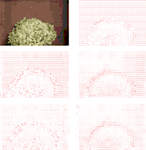Figure 8. Hydrangea real sequence results. First row: the first image of the sequence (left) and the vector-coded ground truth (right). Second row: the optical flow corresponding to L2HS (left) and L1HS (right). Third row: the optical flow corresponding to L2L2 (left) and L1L1 (right).

TABLE 4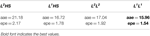Table 4. Performance of L2HS, L1HS, L2L2, and L1L1 algorithms on the Hydrangea real sequence.

Figure 9 compares visually the results of L1L1 (proposed method) and L2L2 (Mitiche et al., 2015) using the different examples in Section 1. The first column depicts the results of the proposed L1L1 method, whereas the second shows the results of Mitiche et al. (2015) (L2L2). The first row shows anaglyphs, the second color-coded depth, and the third 3D object reconstructions. The last two rows displayed both 3D scene flow and projected 2D optical flow fields. We can see that the results are better with L1L1 than with L2L2: the 3D parameters are better defined, clearer, and sharper, especially on the boundaries; flow fields are more regular and smooth.

FIGURE 9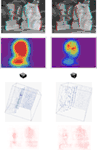Figure 9. Visual inspection of the results of the proposed L1L1 method (first column) and the L2L2 method in Mitiche et al. (2015) (second column). The first row shows anaglyphs, the second color-coded depth, and the third 3D object reconstructions. The last two rows depict 3D scene flow and projected 2D optical flow fields.

Tables 5 and 6 report quantitative comparisons of the four algorithms (L2HS and L1HS in Table 5; L2L2 and L1L1 in Table 6) using three standard error measures (Baker et al., 2011): average angular error (aae), standard angular error (stae), and endpoint error (epe). Errors are computed between the motion resulting from scene flow and the optical flow ground truth. As we do not have scene flow ground truth for these real examples, evaluations of the resulting (projected) optical flow are a good indirect way to assess scene flow results. We constructed an optical flow ground truth using SURF-based (Bay et al., 2008) detection and correspondences of the key points in the two images. Then, the velocity coordinates of these key points are computed, yielding an optical flow ground truth. Tables 5 and 6 confirm that the use of our L1 regularization improves the results and that the L1L1 formulation outperforms the other methods.

TABLE 5
TABLE 6

In the following part of our comparative analysis, we will focus on the assessment (both qualitative and quantitative) of motion within objects boundaries, as preserving these is an important feature of our L1 formulation.

Let us start with a qualitative visual inspection by displaying the images of the gradient of motion for each of the four methods (L2HS, L1HS, L2L2, and L1L1); see Figure 10. We note that with our L1 regularization, points on the boundaries of motion are brighter (sharper). This is due to the fact that our L1 formulation preserves sharp boundaries.

FIGURE 10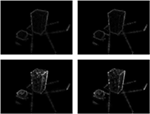Figure 10. Gradients of optical flow for the Marbled blocks sequence. First row: L2HS (left) and L1HS (right). Second row: L2L2 (left) and L1L1 (right).

To support these results, we added quantitative evaluations based on aae, stae, and epe errors: Table 7 reports the results for L2HS and L1HS; Table 8 reports the results for L2L2 and L1L1. Errors are computed between the motion projected from the obtained scene flow and the optical flow ground truth within 7 × 7 windows centered at a set of key points on motion boundaries (the key points are those for which we have motion ground truth). Tables 7 and 8 report the results, which clearly indicate that our L1L1 formulation outperforms all the other methods.

TABLE 7
TABLE 8

6. Conclusion

This study investigated a boundary-preserving method for joint recovery of scene flow and relative depth from a monocular sequence of images. The scheme built upon the basic formulation of Mitiche et al. (2015). It minimized a functional composed of the data conformity term of Mitiche et al. (2015), which relates the image sequence spatiotemporal variations to scene flow and depth, and an L1 regularization term, rather than L2 as in Mitiche et al. (2015). Therefore, this afforded a boundary-preserving version of the basic formulation. The corresponding non-linear Euler–Lagrange equations were discretized and solved iteratively by a scheme, which solved at each iteration a large-scale sparse system of linear equations in the unknowns of scene flow and depth. The image derivatives were estimated by a variational method with L1 regularization. This also led to an iterative method of resolution, which consisted of solving a large sparse system of linear equations at each iteration. Experiments show that the scheme is sound and efficient. The examples demonstrated the need to regularize scene flow and depth so as to take into account their boundaries, i.e., sharp spatial transitions of scene flow or depth. The results justify extensive further investigations, particularly concerning quantitative, i.e., ground truth controlled evaluation, motion of large extent, and image noise and resolution in common practical settings.

The choice of a continuous alternating optimization scheme for our problem can be motivated by two important facts. First, we are dealing with continuous variables, and therefore, a continuous (not discrete) Euler–Lagrange regularization approach is a natural choice. Furthermore, our alternating scheme for solving the ensuing non-linear Euler–Lagrange equations has a computational complexity that behaves linearly w.r.t the number of grid points (N). This is important in practice, particularly when dealing with large image sequences. Of course, it would be very interesting to investigate other regularization options for estimating scene flow and depth from a single image sequence, for instance:

• Discrete Markov Random Fields (MRFs): MRFs were previously investigated for optical flow using sub-modular pairwise potentials (Lempitsky et al., 2008). MRF models can benefit from powerful combinatorial optimization techniques such as graph cuts (Boykov et al., 2001). It is worth noting, however, that adapting discrete MRFs to our continuous setting requires some technical care and is not straightforward.

• Regularization based on non-local means filtering (Favaro, 2010). Non-local means can preserve edges and textures. They were applied successfully to thin structures in depth-from-defocus problems (Favaro, 2010).

• State-of-the-art solvers for non-smooth problems such as the primal-dual algorithm of Chambolle and Pock (2011).

Author Contributions

Ms. YM is a PhD student at INRS under the supervision of Prof. AM. Dr. IA is a professor at École de Technologie Supérieure, Montreal, and co-supervisor of YM. The authors have all contributed, to varying degrees, to the theoretical/methodological/experimental aspects of this paper.

Conflict of Interest Statement

The authors declare that the research was conducted in the absence of any commercial or financial relationships that could be construed as a potential conflict of interest.

References

Aubert, G., Deriche, R., and Kornprobst, P. (1999). Computing optical flow via variational techniques. SIAM J. Appl. Math. 60, 156–182. doi: 10.1137/S0036139998340170

Aubert, G., and Kornprobst, P. (2002). Mathematical Problems in Image Processing. Springer.

Baker, S., Scharstein, D., Lewis, J. P., Roth, S., Black, M. J., and Szeliski, R. (2011). A database and evaluation methodology for optical flow. Int. J. Comput. Vis. 92, 1–31. doi:10.1007/s11263-010-0390-2

Bay, H., Ess, A., Tuytelaars, T., and Van Gool, L. (2008). Speeded-up robust features (surf). Comput. Vision Image Understand. 110, 346–359. doi:10.1016/j.cviu.2007.09.014

Boykov, Y., Veksler, O., and Zabih, R. (2001). Fast approximate energy minimization via graph cuts. IEEE Trans. Pattern Anal. Mach. Intell. 23, 1222–1239. doi:10.1109/34.969114

Chambolle, A., and Pock, T. (2011). A first-order primal-dual algorithm for convex problems with applications to imaging. J. Math. Imag. Vision 40, 120–145. doi:10.1007/s10851-010-0251-1

Ciarlet, P. (1994). Introduction a l’analyse numerique matricielle et a l’optimisation, 5th Edn. Masson.

Debrunner, C., and Ahuja, N. (1998). Segmentation and factorization-based motion and structure estimation for long image sequences. IEEE Trans. Pattern Anal. Mach. Intell. 20, 206–211. doi:10.1109/34.659941

Favaro, P. (2010). “Recovering thin structures via nonlocal-means regularization with application to depth from defocus,” in IEEE Conference on Computer Vision and Pattern Recognition (CVPR), 1133–1140.

Forsythe, G., Malcolm, M., and Moler, C. (1977). Computer Methods for Mathematical Computations. Prentice-Hall.

Heinrich, M. P., Jenkinson, M., Bhushan, M., Matin, T., Gleeson, F. V., Brady, M., et al. (2012). Mind: modality independent neighbourhood descriptor for multi-modal deformable registration. Med. Image Anal. 16, 1423–1435. doi:10.1016/j.media.2012.05.008

Horn, B., and Schunk, B. (1981). Determining optical flow. Artif. Intell. 17, 185–203. doi:10.1016/0004-3702(81)90024-2

Huguet, F., and Devernay, F. (2007). “A variational method for scene flow estimation from stereo sequences,” in 2007 IEEE 11th International Conference on Computer Vision (ICCV) (IEEE), 1–7.

Lempitsky, V. S., Roth, S., and Rother, C. (2008). “FusionFlow: discrete-continuous optimization for optical flow estimation,” in IEEE Conference on Computer Vision and Pattern Recognition (CVPR), 1–8.

Longuet-Higgins, H., and Prazdny, K. (1981). The interpretation of a moving retinal image. Proc. R. Soc. Lond. B 208, 385–397. doi:10.1098/rspb.1980.0057

Marshall, S. (2005). Depth dependence of ambient noise. IEEE J. Oceanic Eng. 30, 275–281. doi:10.1109/JOE.2005.850876

Mitiche, A., and Aggarwal, J. (2013). Computer Vision Analysis of Image Motion by Variational Methods. Springer.

Mitiche, A., Mathlouthi, Y., and Ben Ayed, I. (2015). Monocular concurrent recovery of structure and motion scene flow. Front. ICT 2:16. doi:10.3389/fict

Mitiche, A., and Sekkati, H. (2006). Optical flow 3D segmentation and interpretation: a variational method with active curve evolution and level sets. IEEE Trans. Pattern Anal. Mach. Intell. 28, 1818–1829. doi:10.1109/TPAMI.2006.232

Periaswamy, S., and Farid, H. (2006). Medical image registration with partial data. Med. Image Anal. 10, 452–464. doi:10.1016/j.media.2005.03.006

Pons, J.-P., Keriven, R., and Faugeras, O. (2007). Multi-view stereo reconstruction and scene flow estimation with a global image-based matching score. Int. J. Comput. Vis. 72, 179–193. doi:10.1007/s11263-006-8671-5

Sekkati, H., and Mitiche, A. (2007). A variational method for the recovery of dense 3D structure from motion. J. Rob. Auton. Syst. 55, 597–607. doi:10.1016/j.robot.2006.11.006

Stoer, J., and Bulirsch, R. (2002). Introduction to Numerical Analysis, Texts in Applied Mathematics, 3rd Edn, Vol. 12. New York: Springer.

Sun, D., Roth, S., and Black, M. J. (2010). “Secrets of optical flow estimation and their principles,” in 2010 IEEE Conference on Computer Vision and Pattern Recognition (CVPR) (IEEE), 2432–2439.

Vedula, S., Rander, P., Collins, R., and Kanade, T. (2005). Three-dimensional scene flow. IEEE Trans. Pattern Anal. Mach. Intell. 27, 475–480. doi:10.1109/TPAMI.2005.63

Vogel, C., Schindler, K., and Roth, S. (2013). “Piecewise rigid scene flow,” in IEEE International Conference on Computer Vision (ICCV).

Vogel, C. R. (2002). Computational Methods for Inverse Problems. SIAM Frontiers in Applied Mathematics.

Wedel, A., Rabe, C., Vaudrey, T., Brox, T., Franke, U., and Cremers, D. (2008). Efficient Dense Scene Flow from Sparse or Dense Stereo Data. Springer.

Keywords: scene flow, image sequence analysis, 3D motion, depth, L1 regularization, image derivatives

Citation: Mathlouthi Y, Mitiche A and Ben Ayed I (2016) Monocular, Boundary-Preserving Joint Recovery of Scene Flow and Depth. Front. ICT 3:21. doi: 10.3389/fict.2016.00021

Received: 06 April 2016; Accepted: 15 September 2016;
Published: 30 September 2016

Edited by:

Kaleem Siddiqi, McGill University, Canada

Reviewed by:

K. C. Santosh, University of South Dakota, USA
Scott P. McCloskey, Honeywell, USA

Copyright: © 2016 Mathlouthi, Mitiche and Ben Ayed. This is an open-access article distributed under the terms of the Creative Commons Attribution License (CC BY). The use, distribution or reproduction in other forums is permitted, provided the original author(s) or licensor are credited and that the original publication in this journal is cited, in accordance with accepted academic practice. No use, distribution or reproduction is permitted which does not comply with these terms.

*Correspondence: Amar Mitiche, mitiche@emt.inrs.ca